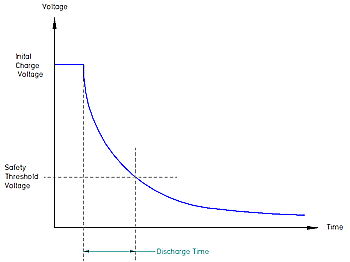Related Resources: capacitance discharge

### Capacitance Discharge Calculator

Electronics and Instrumentation

The following  provides equations and a calculator for determining capacitance discharge rate of a capacitor at known voltage and charge.

Vc = Vo, Q = CVo,

Initial Current, I = Vo/R

Where, Vc = Voltage, I Current and Q = charge

Capacitor Voltage = Q/c =VCapacitance Discharge Calculator ENTER: Capacitor Value (uF) The value of capacitance to be discharged. If there is a wide tolerance, enter the maximum value. ENTER: Initial Charge Voltage (V) The voltage to which the capacitance is initially charged. ENTER: Safety Threshold Voltage (V) The voltage threshold at which the capacitance is considered safely discharged. A typical value is 50V. EITHER ENTER: Resistance Value (ohms) The Ohmic value of the bleed resistor. A bleed resistar at this ohmic value will ensure safe discharge within 0.0150627690651412 s The initial power in the resistor is W and the time constant of the discharge is s The total energy discharged is J OR ENTER: Discharge Time (s) The time taken to reach safe discharge condition. To ensure safe discharge within this time the bleed resistor should be at or below ohms The initial power in the resistor is W and the time constant of the discharge is s The total energy discharged is J